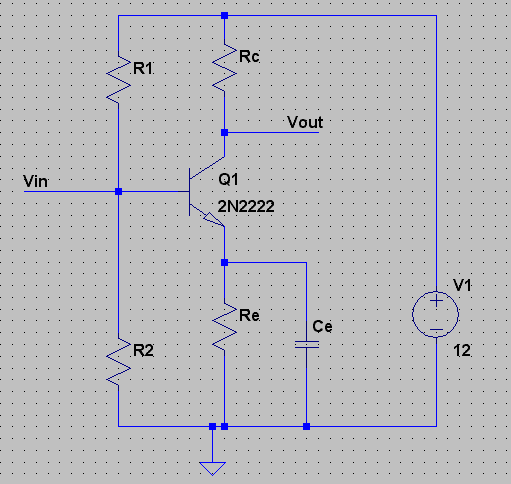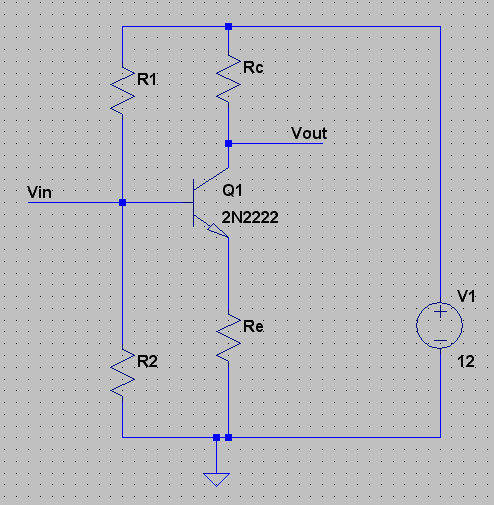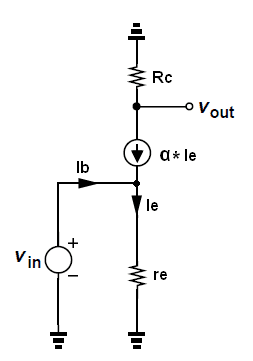# Voltage gain independent of transistor?

Gold Member
My professor asked me to provide a circuit design for a voltage divider biasing circuit whereby the transistor beta is independent of the voltage gain, which he wants to be -100.

I maintain this is impossible, as Ic = B*Ib, changing the beta obviously effects the gain.

Right?

Gold Member
I have notes on how, we can make the voltage divider behave as a constant voltage supply - by making the current through the divider resistors large in comparison to Ib.

This makes sense, but still, if we change the transistor and beta, then Ib is amplified by a new beta, this effects Ic and all the voltages across the transistor (C to E), the collector resistor, and so on....

He maintains that I1 = I2+Ib
Vbb = Vcc - I1*R1 = Vcc - (Ib+I2)*R1
So, Vbb is dependent on Ib, which we do not want

So we make Ib very small, and Vbb is approx. equal to Vcc - I2*R1
If beta is much larger than 1, Ic is approx equal to B*Ie equal to B*Ic

Vbb - Vbe - Ic*Re = 0
Ic = Vbb - Vbe divided Re.
Now Ic is independent of Beta.

Can some one explain how Ic is independent of beta? it simply doesn't make sense.

2021 Award
FOIWATER said:
the transistor beta is independent of the voltage gain
I think you meant “the voltage gain is independent of the transistor beta”.

Total independence is not really possible with a single transistor circuit. But, if the collector resistor is 100 times the value of the emitter resistor then base voltage will be amplified by -100 and appear across the collector resistor. There will be a very slight gain error due to variation of beta.

Gold Member
yes sorry thats what i meant

Im not really understanding,

Gold Member
I dont even see how it's remotely possible.

I designed the circuit using firm rules provided by a textbook,

and I got my biasing point, then during small signal analysis, I calculated ib, I calculated ic, and I find the voltage gain

Then, if I double beta, I double ic and I double the voltage gain

Everytime

Gold Member
I think I have come to a conclusion,

for small values of ib, Vbb is relatively constant.

Ib = Vcc - Vbe/(Re)*(1+beta)

increasing beta here, will certainly decrease Ib,

but increasing beta will decrease the dynamic resistance of the junction,

and if the circuit is properly biased this could lead to an increasing beta and a decreasing Ib, leading to a constant small signal collector current and therefore constant voltage gain.

Any one know if this makes sense?

Gold Member
baluncore - I appreciate the help

I am doing it, too.

pls don't mistake my frustration for lack of motivation

Gold Member
To a first order the gain of a common emitter amplifier is Rc/Re. Changes in beta will have small effects for small signal applications. (for example a different drop across Rb due to change in Ib)

Gold Member
I have designed a circuit and the calculated gain is -100.
But Rc/Re is only about 4 or 5
it is common emitter, voltage divider biased.
On paper, it works great. different transistors don't effect the gain because Ib is very small, and Vbb is approx. constant.
So as beta doubles, Ib = Vbb - 0.7/ (1+b)*Re approx. halves. But that keeps Ic the same.

On paper it works, but then I ran it through spice and got some distortion for the requries 0.02v input.
works fine for 0.002v input, I get that proper -100 gain, and a swing between almost exactly -0.2 and +0.2 volts

I attached the waveforms and cct

note the distortion for 0.02v

(I assume it's the input causing it?)

#### Attachments

Gold Member
I tried re designing this for Vce = 6 volts (middle of the load line)

and it was even worse.

Is it possible distortion is simply inherent to this circuit at such a high signal voltage?

Jony130
Your circuit is example of a bad circuit design. And also you demand too much from this simple circuit.
CE amplifier don't like driving low load resistances.
To improve a circuit you need to add some negative feedback (remove Ce capacitor).Or add another BJT (emitter follower).

Last edited:
Gold Member
but the Ce capacitor is what gives the high gain?

the gain of this circuit is inversely related to Re. and unless it's bypassed the gain would be extremely low.

Jony130
You must understand that this simple CE amplifier cannot provide at the same time high gain and low distortion and drive the low resistive load (Rc should always be smaller then RL). This is why in real life we don't use this simple circuit. Here you have a good design amplifier.

#### Attachments

Last edited:
willem2
The problem here is that for small signals with enough frequency, the capacitor at the emitter will look like a short.

with no input, the base current will be

$$I_b = \frac {V_0 - 0.7} {\beta R_e}$$

where V_0 is the bias voltage set by R1 and R2.

but for small signals, the base current is set by the exponential relation between voltage and current of the base-emitter junciton

$$i_b = I_b (e^{\frac {v}{V_T}}-1)$$

v is the small signal voltage: V_in
and i_b the small signal base current

where V_T, the thermal voltage is about 26 mV at room temperature.

you can replace

$$e^{\frac {v}{V_T}}-1$$ with v/V_T only if v is a lot smaller than V_T, and
only then can you expect linear amplification.

The amplifier will have a low input impedance at signal frequencies, It wouldn't surprice me if that would produce a low pass filter together with C1. Try and see what the gain is with 0.002 V input at 10 Khz

Gold Member
i'll try it out willem thanks

Jony yeah I understand what you are saying.

It cannot do both.

Gold Member
so what happens if I use a bigger load

I notice in spice, the higher resistance load I use, the more toward +2 volts the waveform approaches, but the further negative the negative half cycle goes almost to -3 volts.

Jony130
To improve circuit linearity you need to use negative feedback. There is no other way.

Gold Member
I understand, I am asking what is causing this phenomenon

Jony130
The voltage gain is a signal level dependent.

Av = gm * Rc||RL ≈ 40*Ic * Rc||RL.

When the input signal swings positive so that the collector current increases, the voltage gain also increase his value. And for negative input signal swings the collector current drops. So the gain decrease his value. As you can see the voltage gain of this CE amplifier was changing when the output signal has swinging. This represents a high level of distortion.

Gold Member
but it's clipping right?

I guess that's the other tradeoff.

What is it about Vce being low that causes the clipping.

I can't come up with a good explanation for it.

Is it because the transistor is essentially saturation on the peaks of the wave?

About the explanation you provided for the gain.. thanks I didn't think of it that way

Last edited:
Gold Member
When I said Rc/Re sets the gain it assumed there was no bypass capacitor across Re and no load resistor. With a bypass capacitor the gain increases towards infinity with frequency because Re effectively becomes 0. The load resistor has a gain effect also.

If you want a gain of 100, use Rc = 10K and Re = 100 and no capacitor across Re and no Rload. Then you will see how the simple common emitter amp really works. Vary Re and see how the gain changes at the collector. Notice what happens as you approach the limit caused by Beta.

Once you understand that simple circuit you can experiment with the effect of Ce and Rload and how they change the operation.

Gold Member
but the gain here is calculated as -gm*Rl||Rc , which isn't dependent on Re. another way to express it would be gain = -beta*Rl||Rc / (Rpi + (1+B)*Re) if Re goes to zero the gain is high but not infinity it is proportional to 1/Rpi.

Unless I missed what you were trying to say?

Gold Member
Yes, you missed what I was trying to say, but maybe I missed what you are trying to understand. Do you understand Rc/Re in the simple no load common emitter circuit? Is the example I stated in post 23 trivial for you to understand? Do you fully understand what Re does in that simple circuit?

Gold Member
I tried calculating the gain for a simple common emitter, and assuming beta is large and Rpi is << Re, I got -Rc/Re.
Is this correct?
If by do I fully understand what Re does, you mean do I have a feel for it beyond this calculation, then no not really. I see how the ratio effects the gain for an unloaded circuit though.

I Also assume here, changing beta would in fact effect the gain. although the equation for gain doesn't seem to show it?

Gold Member
I Also assume here, changing beta would in fact effect the gain. although the equation for gain doesn't seem to show it?

The basic calculation you performed assumes constant Vbe, which of course is an approximation for small signal models.

Now you can see how the small signal gain of a transistor amplifier can be largely independent of Beta. But of course there are secondary effects that modify the gain based on other transistor parameters. look at the models on this page.

http://en.wikipedia.org/wiki/Bipolar_junction_transistor

Especially descriptive are the unapproximated Ebers–Moll equations which are largely concerned with Vbe/Vt and reverse saturation currents.

Jony130
Let my try to clarify some confusion that arise here about CE stage voltage gain.

First this circuit.Ce capacitor shorts Re resistor for the AC signal. So we have no negative feedback anymore for AC signal.

And the voltage gain of this stage is equal to

$\Large\frac{Vout}{Vin} = -gm*Rc *\frac{\beta}{\beta +1} = -\frac{Rc *\beta}{re *(\beta+1)}\approx-\frac{R_C}{re}$

where re is equally to

$\Large re = \frac{Vt}{Ie} = \frac{\beta}{gm(\beta+1)} \approx \frac{1}{gm} \approx \frac{26mV}{Ic}$

Now if we remove Ce capacitor from the circuitThe voltage gain will now be equal to

$\Large \frac{Vout}{Vin} = -\frac{Rc}{re + Re} *\frac{\beta}{(\beta+1)} \approx-\frac{R_C}{(re + R_E)}\approx -\frac{R_C}{R_E}$

Ss you can see beta would in fact effect the gain by the factor of β/(β + 1).
For the Av = 100 and β = 500 we have

A = 100*500/501 = 99.8V/V

But if we have a BJT with β = 20 the voltage gain will drop also but not much.

A = 100 * 20/21 = 95.24V/V

Also high beta BJT will have a smaller ro = dVce/dIc = (Va - Vce)/Ic value. So this will also affect the gain.

Va = Early voltage

#### Attachments

Last edited:
Gold Member
Jony shouldn't Vo = -Beta*ib*Rc in your circuit?
And Vo/Vs = -gm*Rc ? I am not sure where B+1 is coming from in the denominator, as Re is shorted? also the Beta in the numerator. I had one there initially, but it was used to express gm as gm = Beta/Rpi

Last edited:
Jony130
Yes you right, I made a simply error in the voltage gain equation.
The voltage gain for CE amplifier with Ce capacitor is of-course equal to

$\Large Av = \frac{Vout}{Vin} = - gm*Rc = -\frac{Rc}{re} * \frac{\beta}{(\beta+1)}\approx -\frac{Rc}{re}$

This betas in equation is here because of a re - small signal resistance beetwen base and emitter looking into the emitter

re = dVbe/dIe = Vt/Ie ≈ 26mV/Ic

and since gm = Ic/Vt

and Ic = alpha*Ie = (β/(β + 1))*Ie we get

gm = Ic/Vt = Ic/Ie * Ie/Vt = Ic/Ie * 1/re = β/(β+1)*1/re

Also we can use small-signal T model to find out the voltage gain.So we have

Vout = - alpha*Ie*Rc and Vin = Ie*re

So next we have

Av = Vout/Vin = (- alpha*Ie*Rc)/(Ie*re) = Rc/re * alpha = Rc/re * β/(β + 1) ≈Rc/re

Also notice that some professors use Rpi = dVbe/dIb instead of re.

Rpi = (β + 1)*re

Av = (β*Rc)/Rpi = (β*Rc)/( (β + 1)*re) = Rc/re * β/(β + 1) looks familiar ?

But as you can see re gives simpler equation and better inside.
https://www.physicsforums.com/showpost.php?p=4061337&postcount=54

P.S
I purposely omitted the minus sign in the gain equations as as unimportant.

Last edited: